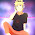### leetcode Question 72: Populating Next Right Pointers in Each Node

Populating Next Right Pointers in Each Node

Given a binary tree
    struct TreeLinkNode {
}

Populate each next pointer to point to its next right node. If there is no next right node, the next pointer should be set to NULL.
Initially, all next pointers are set to NULL.
Note:
• You may only use constant extra space.
• You may assume that it is a perfect binary tree (ie, all leaves are at the same level, and every parent has two children).
For example,
Given the following perfect binary tree,
         1
/  \
2    3
/ \  / \
4  5  6  7

After calling your function, the tree should look like:
         1 -> NULL
/  \
2 -> 3 -> NULL
/ \  / \
4->5->6->7 -> NULL

Idea is to use dfs and only constant space is used. The key is to use the "next" pointer and search right child first then the left child. Details see the code comment.

Code:
/**
* Definition for binary tree with next pointer.
* struct TreeLinkNode {
*  int val;
*  TreeLinkNode *left, *right, *next;
*  TreeLinkNode(int x) : val(x), left(NULL), right(NULL), next(NULL) {}
* };
*/
class Solution {
public:
if (!root){return;}  // if current node is not valid return

if (root->next==NULL){ // if current node is not in the right boundary of this level
if (root->right){root->right->next = NULL;} // if has right child, its next is null
if (root->left){root->left->next = root->right;}//if has left child, its next is its right
}else{ // if the current node has next node in its right
TreeLinkNode* p = root->next; //the pointer travle along this level
TreeLinkNode* q=NULL; // the next valid pointer in the level+1 , or NULL if not found
while (p){ //find the next valid child of root node
if (p->left){q =p->left; break;}
if (p->right){q =p->right; break;}
p=p->next;
}
if (root->right){root->right->next = q;} //set right child if exists
if (root->left && root->right ){root->left->next = root->right;}//set left if right exists
if (root->left && !root->right) {root->left->next = q;} // set left if right not exist
}

dfs(root->right); // search right child, order is important
dfs(root->left);  // search left child
}
void connect(TreeLinkNode *root) {
// Start typing your C/C++ solution below
// DO NOT write int main() function
if (!root){return;}
root->next = NULL;
dfs(root);
}
};


Old solution (BFS):
We know that the tree is full binary tree, which is a very powerful condition that we can use.
As can be seen in the tree above, we need to deal with the nodes according to each level of the tree.
Easily the level-order traversal popped out!
What we need? Yes, just a queue!
What about the level?  Here comes the condition of this problem. A full binary tree which have  ith level have
2^i - 1 nodes.

Once we have the queue, the problem is quite easy. Just make the last node's next pointer NULL, set other nodes' next to the next element in the queue. Well done!

The space needed is less than 2^i-1;

Code:
/**
* Definition for binary tree with next pointer.
* struct TreeLinkNode {
*  int val;
*  TreeLinkNode *left, *right, *next;
*  TreeLinkNode(int x) : val(x), left(NULL), right(NULL), next(NULL) {}
* };
*/
class Solution {
public:
void connect(TreeLinkNode *root) {
// Start typing your C/C++ solution below
// DO NOT write int main() function
if (root==NULL) {return;}
que.push(root);
int i=1;
int l=1;
while (!que.empty()){
que.pop();
if ((p->right!=NULL)&&(p->left!=NULL)){
que.push(p->left);
que.push(p->right);
}
if (i==(pow(2,l)-1)){
p->next = NULL;
i++;
l++;
}else{
p->next = que.front();
i++;
}
}

}
};


1.I have a different solution, which looks neater, please check out the following code. It uses recursive call, not sure about time complexity compared to yours.

void connect(TreeLinkNode *root) {
if(!root)
return;

TreeLinkNode* l = root->left;
TreeLinkNode* r = root->right;
if(l && r) {
l->next = r;

while(l->right) {
l = l->right;
r = r->left;
l->next = r;
}

connect(root->left);
connect(root->right);
}

1.Recursive methods won't use only constant space.

2.Also, in the first dfs function, if you change the order of dfs(root->right) and dfs(root->left), the code also works fine and pass the test.

3.you can consider the solution here:

public class Solution {
public void connect(TreeLinkNode root) {

while(root != null){